### Home > CALC > Chapter 3 > Lesson 3.3.2 > Problem3-103

3-103.
1.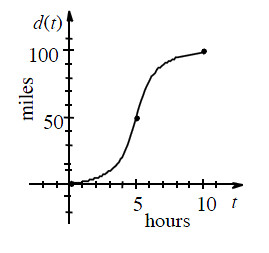The graph at right records the distance a bicyclist travels from Oshkosh to a town 10 miles away. Homework Help ✎

1. Describe the velocity of the bicyclist.

2. What was the bike's average velocity

3. Approximate the bike's instantaneous velocity at t = 5 hours.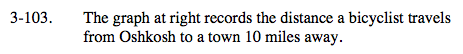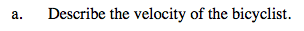The velocity is the slope of a distance graph.

The velocity is always positive. The bicyclist accelerates between t = 0 and t = 5 and then deccelerates between t = 5 and t = 10. S/he is moving fastest at t = 5.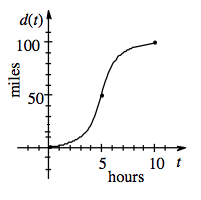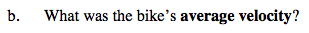$\text{Average velocity between }t=0 \text{ and }t=10=\frac{\Delta \text{distance}}{\Delta \text{time}}=\frac{d(10)-d(0)}{10-0}$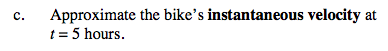IROC at a point = slope of the tangent line at that point.